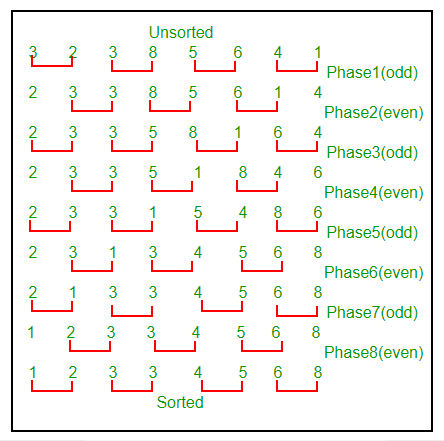# Odd-Even Sort / Brick Sort

• Difficulty Level : Easy
• Last Updated : 28 Jun, 2021

This is basically a variation of bubble-sort. This algorithm is divided into two phases- Odd and Even Phase. The algorithm runs until the array elements are sorted and in each iteration two phases occurs- Odd and Even Phases.
In the odd phase, we perform a bubble sort on odd indexed elements and in the even phase, we perform a bubble sort on even indexed elements.

Attention reader! Don’t stop learning now. Get hold of all the important DSA concepts with the DSA Self Paced Course at a student-friendly price and become industry ready.  To complete your preparation from learning a language to DS Algo and many more,  please refer Complete Interview Preparation Course.

In case you wish to attend live classes with experts, please refer DSA Live Classes for Working Professionals and Competitive Programming Live for Students.

## C++

 `// A C++ Program to implement Odd-Even / Brick Sort``#include``using` `namespace` `std;` `// A function to sort the algorithm using Odd Even sort``void` `oddEvenSort(``int` `arr[], ``int` `n)``{``    ``bool` `isSorted = ``false``; ``// Initially array is unsorted` `    ``while` `(!isSorted)``    ``{``        ``isSorted = ``true``;` `        ``// Perform Bubble sort on odd indexed element``        ``for` `(``int` `i=1; i<=n-2; i=i+2)``        ``{``            ``if` `(arr[i] > arr[i+1])``             ``{``                ``swap(arr[i], arr[i+1]);``                ``isSorted = ``false``;``              ``}``        ``}` `        ``// Perform Bubble sort on even indexed element``        ``for` `(``int` `i=0; i<=n-2; i=i+2)``        ``{``            ``if` `(arr[i] > arr[i+1])``            ``{``                ``swap(arr[i], arr[i+1]);``                ``isSorted = ``false``;``            ``}``        ``}``    ``}` `    ``return``;``}` `// A utility function ot print an array of size n``void` `printArray(``int` `arr[], ``int` `n)``{``   ``for` `(``int` `i=0; i < n; i++)``       ``cout << arr[i] << ``" "``;``   ``cout << ``"\n"``;``}` `// Driver program to test above functions.``int` `main()``{``    ``int` `arr[] = {34, 2, 10, -9};``    ``int` `n = ``sizeof``(arr)/``sizeof``(arr);` `    ``oddEvenSort(arr, n);``    ``printArray(arr, n);` `    ``return` `(0);``}`

## Java

 `// Java Program to implement``// Odd-Even / Brick Sort``import` `java.io.*;` `class` `GFG``{``    ``public` `static` `void` `oddEvenSort(``int` `arr[], ``int` `n)``    ``{``        ``boolean` `isSorted = ``false``; ``// Initially array is unsorted` `        ``while` `(!isSorted)``        ``{``            ``isSorted = ``true``;``            ``int` `temp =``0``;` `            ``// Perform Bubble sort on odd indexed element``            ``for` `(``int` `i=``1``; i<=n-``2``; i=i+``2``)``            ``{``                ``if` `(arr[i] > arr[i+``1``])``                ``{``                    ``temp = arr[i];``                    ``arr[i] = arr[i+``1``];``                    ``arr[i+``1``] = temp;``                    ``isSorted = ``false``;``                ``}``            ``}` `            ``// Perform Bubble sort on even indexed element``            ``for` `(``int` `i=``0``; i<=n-``2``; i=i+``2``)``            ``{``                ``if` `(arr[i] > arr[i+``1``])``                ``{``                    ``temp = arr[i];``                    ``arr[i] = arr[i+``1``];``                    ``arr[i+``1``] = temp;``                    ``isSorted = ``false``;``                ``}``            ``}``        ``}` `        ``return``;``    ``}``    ``public` `static` `void` `main (String[] args)``    ``{``        ``int` `arr[] = {``34``, ``2``, ``10``, -``9``};``        ``int` `n = arr.length;` `        ``oddEvenSort(arr, n);``        ``for` `(``int` `i=``0``; i < n; i++)``            ``System.out.print(arr[i] + ``" "``);` `        ``System.out.println(``" "``);``    ``}``}``// Code Contribute by Mohit Gupta_OMG <(0_o)>`

## Python3

 `# Python Program to implement``# Odd-Even / Brick Sort` `def` `oddEvenSort(arr, n):``    ``# Initially array is unsorted``    ``isSorted ``=` `0``    ``while` `isSorted ``=``=` `0``:``        ``isSorted ``=` `1``        ``temp ``=` `0``        ``for` `i ``in` `range``(``1``, n``-``1``, ``2``):``            ``if` `arr[i] > arr[i``+``1``]:``                ``arr[i], arr[i``+``1``] ``=` `arr[i``+``1``], arr[i]``                ``isSorted ``=` `0``                ` `        ``for` `i ``in` `range``(``0``, n``-``1``, ``2``):``            ``if` `arr[i] > arr[i``+``1``]:``                ``arr[i], arr[i``+``1``] ``=` `arr[i``+``1``], arr[i]``                ``isSorted ``=` `0``    ` `    ``return`  `arr ``=` `[``34``, ``2``, ``10``, ``-``9``]``n ``=` `len``(arr)` `oddEvenSort(arr, n);``for` `i ``in` `range``(``0``, n):``    ``print``(arr[i], end ``=` `' '``)``    ` `# Code Contribute by Mohit Gupta_OMG <(0_o)>`

## C#

 `// C# Program to implement``// Odd-Even / Brick Sort``using` `System;` `class` `GFG``{``    ``public` `static` `void` `oddEvenSort(``int` `[]arr, ``int` `n)``    ``{``        ``// Initially array is unsorted``        ``bool` `isSorted = ``false``;` `        ``while` `(!isSorted)``        ``{``            ``isSorted = ``true``;``            ``int` `temp =0;` `            ``// Perform Bubble sort on``            ``// odd indexed element``            ``for` `(``int` `i = 1; i <= n - 2; i = i + 2)``            ``{``                ``if` `(arr[i] > arr[i+1])``                ``{``                    ``temp = arr[i];``                    ``arr[i] = arr[i+1];``                    ``arr[i+1] = temp;``                    ``isSorted = ``false``;``                ``}``            ``}` `            ``// Perform Bubble sort on``            ``// even indexed element``            ``for` `(``int` `i = 0; i <= n - 2; i = i + 2)``            ``{``                ``if` `(arr[i] > arr[i+1])``                ``{``                    ``temp = arr[i];``                    ``arr[i] = arr[i+1];``                    ``arr[i+1] = temp;``                    ``isSorted = ``false``;``                ``}``            ``}``        ``}``        ``return``;``    ``}``    ` `    ``// Driver code``    ``public` `static` `void` `Main ()``    ``{``        ``int` `[]arr = {34, 2, 10, -9};``        ``int` `n = arr.Length;``        ` `        ``// Function calling``        ``oddEvenSort(arr, n);``        ``for` `(``int` `i = 0; i < n; i++)``            ``Console.Write(arr[i] + ``" "``);` `        ``Console.WriteLine(``" "``);``    ``}``}` `// This code is contributed by Sam007`

## Javascript

 ``

Output :

`-9 2 10 34 `

We demonstrate the above algorithm using the below illustration on the array = {3, 2, 3, 8, 5, 6, 4, 1}Please refer wiki for proof of correctness.
Time Complexity : O(N2) where, N = Number of elements in the input array.
Auxiliary Space : O(1). Just like bubble sort this is also an in-place algorithm.
Exercise
In our program in each iteration we first do bubble sort on odd indexed elements and then a bubble sort on the even indexed elements.
Will we get a sorted result if we first perform a bubble sort on even indexed element first and then on the odd indexed element ?
References
https://en.wikipedia.org/wiki/Odd%E2%80%93even_sort
This article is contributed by Rachit Belwariar. If you like GeeksforGeeks and would like to contribute, you can also write an article and mail your article to review-team@geeksforgeeks.org. See your article appearing on the GeeksforGeeks main page and help other Geeks.